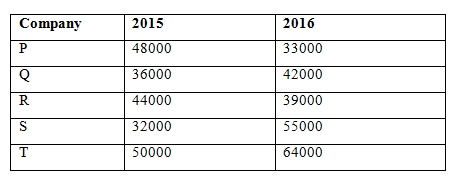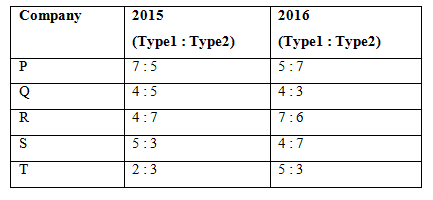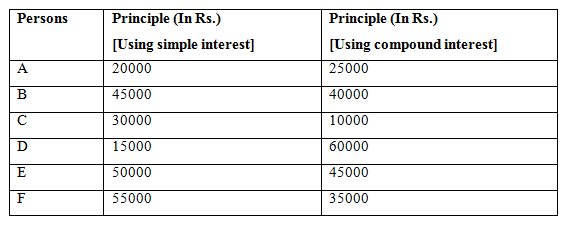# Quantitative Aptitude Questions (Data Interpretation) for RRB Assistant Mains 2018 Day-208

Dear Readers, IBPS is conducting Online Examination for the recruitment of RRB Assistant. To enrich your preparation here we are providing new series of Data Interpretation – Quantitative Aptitude Questions. Candidates those who are appearing in RRB Assistant Mains Exams can practice these Quantitative Aptitude questions daily and make your preparation effective.

[WpProQuiz 3805]

Click “Start Quiz” to attend these Questions and view Solutions

Directions (1 – 5) Study the following information carefully and answer the given questions:

The following table-1 shows the total production of 2 different types of metals in two different years in different companies in a certain state.The table-2 shows the ratio between the total productions of type 1 metals to that of type 2 metals in a certain state.1) Find the ratio between the total production of company P and R together in the year 2015 to that of total production of type 2 metals in company Q, R and S together in the year 2016?

a) 56: 33

b) 14: 9

c) 92: 71

d) 217: 175

e) None of these

2) Find the difference between the total production of company Q and R together in the year 2016 to that of total production of type1 metals of company P, S and T together in the year 2015?

a) 13000

b) 8000

c) 10000

d) 6500

e) None of these

3) Find the average production of type 1 metals in company P, Q and R together in the year 2015?

a) 22000

b) 21000

c) 18500

d) 20000

e) None of these

4) Total production of type 1 metals of company T in the year 2015 is approximately what percentage of total production of type 2 metals of company Q in the year 2016?

a) 95 %

b) 110 %

c) 75 %

d) 125 %

e) 140 %

5) Total production of company P, R and T together in the year 2015 is approximately what percentage more/less than the total production of company Q, S and T together in the year 2016?

a) 12 % less

b) 15 % more

c) 12 % more

d) 15 % less

e) 10 % less

Directions (6 – 10) Study the following information carefully and answer the given questions:

The following table shows the principle amount invested by six persons on simple interest and compound interest.6) If C invested his amount on C.I for 3 years at 5 % per annum and E invested his amount on S.I at 8 % per annum for 4 years, then find the sum of the compound interest of C to that of simple interest of E?

a) Rs. 16528.75

b) Rs. 15242.50

c) Rs. 17576.25

d) Rs. 18527

e) None of these

7) The principle amount invested by A, C and F together in simple interest is approximately what percentage of the principle amount invested by B, D and E together in compound interest?

a) 72 %

b) 85 %

c) 96 %

d) 114 %

e) 60 %

8) Find the difference between the simple interest earned by B after 5 years at 6 % per annum to that of compound interest earned by D after 2 years at 8 % per annum?

a) Rs. 4123

b) Rs. 3516

c) Rs. 2879

d) Rs. 3138

e) None of these

9) The total principle amount invested in simple interest is approximately what percentage of the total principle amount invested in compound interest?

a) 85 %

b) 75 %

c) 50 %

d) 100 %

e) 110 %

10) Find the average simple interest earned by B, C, E and F after 3, 4, 5 and 6 years respectively at 10 % per annum?

a) Rs. 21560

b) Rs. 18525

c) Rs. 20875

d) Rs. 23430

e) None of these

The total production of company P and R together in the year 2015

= > 48000 + 44000 = 92000

The total production of type 2 metals in company Q, R and S together in the year 2016

= > 42000*(3/7) + 39000*(6/13) + 55000*(7/11)

= > 18000 + 18000 + 35000 = 71000

Required ratio = 92000: 71000 = 92: 71

The total production of company Q and R together in the year 2016

= > 42000 + 39000 = 81000

The total production of type1 metals of company P, S and T together in the year 2015

= > 48000*(7/12) + 32000*(5/8) + 50000*(2/5)

= > 28000 + 20000 + 20000 = 68000

Required difference = 81000 – 68000 = 13000

The total production of type 1 metals in company P, Q and R together in the year 2015

= > 48000*(7/12) + 36000*(4/9) + 44000*(4/11)

= > 28000 + 16000 + 16000 = 60000

Required average = 60000/3 = 20000

The total production of type 1 metals of company T in the year 2015

= > 50000*(2/5) = 20000

The total production of type 2 metals of company Q in the year 2016

= > 42000*(3/7) = 18000

Required % = (20000/18000)*100 = 111.11 % = 110 %

The total production of company P, R and T together in the year 2015

= > 48000 + 44000 + 50000 = 142000

The total production of company Q, S and T together in the year 2016

= > 42000 + 55000 + 64000 = 161000

Required % = [(161000 – 142000)/161000]*100 = 11.8 % less

The compound interest of C

= > [10000*(105/100)*(105/100)*(105/100)] – 10000

= > 11576.25 – 10000 = 1576.25

The simple interest of E

= > [50000*8*4/100]

= > 16000

Required sum = 1576.25 + 16000 = Rs. 17576.25

The principle amount invested by A, C and F together in simple interest

= > 20000 + 30000 + 55000 = 105000

The principle amount invested by B, D and E together in compound interest

= > 40000 + 60000 + 45000 = 145000

Required % = (105000/145000)*100 = 72 %

The simple interest earned by B

= > (45000*5*6)/100 = Rs. 13500

The compound interest earned by D

= > [60000*(108/100)*(108/100)] – 60000

= > 69984 – 60000 = Rs. 9984

Required difference = 13500 – 9984 = Rs. 3516

The total principle amount invested in simple interest

= > 20000 + 45000 + 30000 + 15000 + 50000 + 55000 = Rs. 215000

The total principle amount invested in compound interest

= > 25000 + 40000 + 10000 + 60000 + 45000 + 35000 = Rs. 215000

Required % = (215000/215000)*100 = 100 %

The total simple interest earned by B, C, E and F

= > [(45000*10*3)/100] + [(30000*10*4)/100] + [(50000*5*10)/100] + [(55000*10*6)/100]

= > 13500 + 12000 + 25000 + 33000 = Rs. 83500

Required average = 83500/4 = Rs. 20875

Daily Practice Test Schedule | Good Luck

 Topic Daily Publishing Time Daily News Papers & Editorials 8.00 AM Current Affairs Quiz 9.00 AM Quantitative Aptitude “20-20” 11.00 AM Vocabulary (Based on The Hindu) 12.00 PM General Awareness “20-20” 1.00 PM English Language “20-20” 2.00 PM Reasoning Puzzles & Seating 4.00 PM Daily Current Affairs Updates 5.00 PM Data Interpretation / Application Sums (Topic Wise) 6.00 PM Reasoning Ability “20-20” 7.00 PM English Language (New Pattern Questions) 8.00 PM